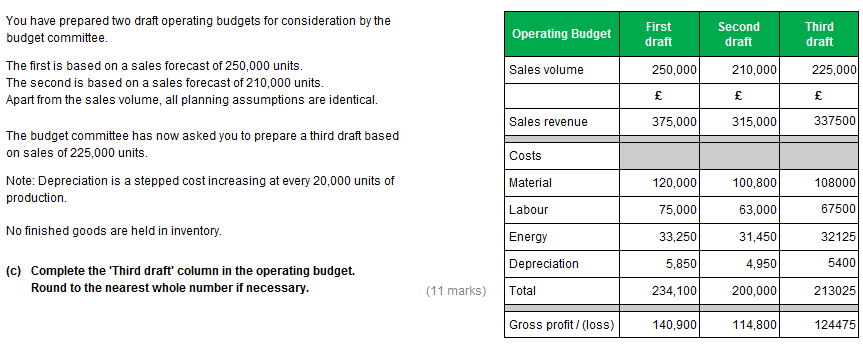# AAT level 4 mabu sample assessment 2 task 5c

Please could somebody attempt to explain how the third draft energy and depreciation figure has been calculated. I know the answer just can't work out how to get there.

Thanks

• Hi,

Is this the one with 1st draft 250K units, 2nd draft 210K units and 3rd draft 225K units?

It mentions that depreciation is a stepped cost increasing every 20,000 units.

Draft 1 - 250,000 units
250,000/20,000 = 12.5 this means its on the 13th step
Depreciation cost of £5850 divide by 13 steps = £450 per step

Check step cost on Draft 2 - 210,000 units
210,000/20,000 = 10.5 means 11th step. 11 steps x £450 per step = £4950 this confirms we are right

Draft 3 - 225,000 units
225,000/20,000 = 11.25 means 12th step x £450 = £5400

It doesn't say what characteristic Energy cost is but after doing a few examples you would expect it to be semi-variable. Use the high/low method.

£33,250-£31,450 / 250,000 - 210,000 = £0.045 (variable cost per unit)

Take 250,000 units for example,
Variable cost = 250,000 x £0.045 = £11,250
Where total cost = £33,250
means Fixed cost = £33,250 - £11,250 = £22,000

You can check this against draft 2 - 210,000 units if you wish to confirm
VC = 210,000 x £0.045 = £9450
Plus FC = £22,000
Total costs = £31,450

Hence for draft 3 - 225,000 units
VC = 225,000 x £0.045 = £10,125
Plus FC = £22,000
Total energy cost = 32,125

I don't have the answers so fingers crossed I have got this correct.

• Thanks @N4T, the calculations are spot on.

My only other question would be, how did you know that energy was a semi variable cost?
• This question is testing you variable cost, fixed cost, stepped cost and semi variable. You had variable cost - the revenue, material and labour. I don't remember if the question involved a fixed cost...usually rent. You were told depreciation was a stepped cost so the assumption would be semi-variable left as it is obviously neither fixed nor variable and can't be another stepped as you will need additional data. It is a process of elimination and common sense. Energy is 90% semi-variable. Pay a standing charge plus usage on top.

The more practice assessments you do the more obvious this becomes, this type of question doesn't change just the figures.
• You can work out that energy is a semi variable cost by first working out the cost per unit in each draft. If it was a variable cost it would be the same unit cost in each draft. If it is different and it doesn't tell you in the question that it is fixed, then you know it is a semi variable cost.

The best approach is to check the unit cost in each draft first. If its the same you can just flex it to the third draft, if its not you will need to use hi-lo method.• Hi Guys,

I was struggling with the Energy part to so thank you for clarifying that.

One other issue with that question is that the total of the costs on the second draft is incorrect to me? Please could somebody clarify this?